Function Repository Resource:

# NetContract

Contract a subset of vertices in a NetGraph or NetChain into a single vertex

Contributed by: Maria Sargsyan
 ResourceFunction["NetContract"][net, {start, end}] contracts all the vertices from the start to the end in a NetGraph or NetChain into a single vertex. ResourceFunction["NetContract"][net, {start, end}→ name] contracts all the vertices from the start to the end in a NetGraph or NetChain into a single named vertex. ResourceFunction["NetContract"][net, string] contracts all the vertices which starts with a name string in a NetGraph or NetChain into a single vertex. ResourceFunction["NetContract"][net, string → name] contracts all the vertices which starts with a name string in a NetGraph or NetChain into a single named vertex. ResourceFunction["NetContract"][net, pattern] contracts all the vertices matched by a pattern in a NetGraph or NetChain into a single vertex. ResourceFunction["NetContract"][net, pattern→name] contracts all the vertices matched by a pattern in a NetGraph or NetChain into a single named vertex. ResourceFunction["NetContract"][net, rule1, rule2 , …] performs all the contractions specified by the rulei….

## Details

The patterns can be only string patterns such as RegularExpression[regex], s1~~ s2 ~~ or pure functions which operate on strings.
In ResourceFunction["NetContract"][net,{start,end}, ], the {start, end} can be any start and end specified in NetTake[net, {start, end}].

## Examples

### Basic Examples (9)

Define a net:

 In:=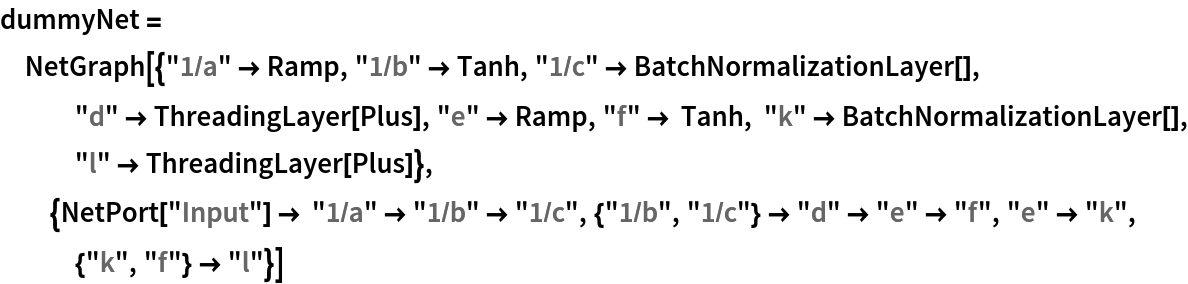Out=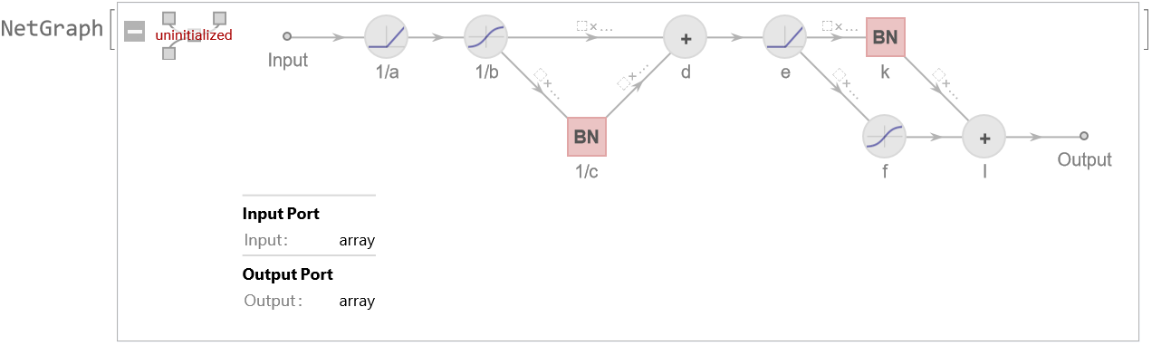Obtain a new net by contracting everything from the starting to the ending node:

 In:=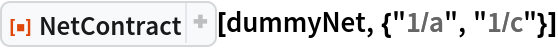Out=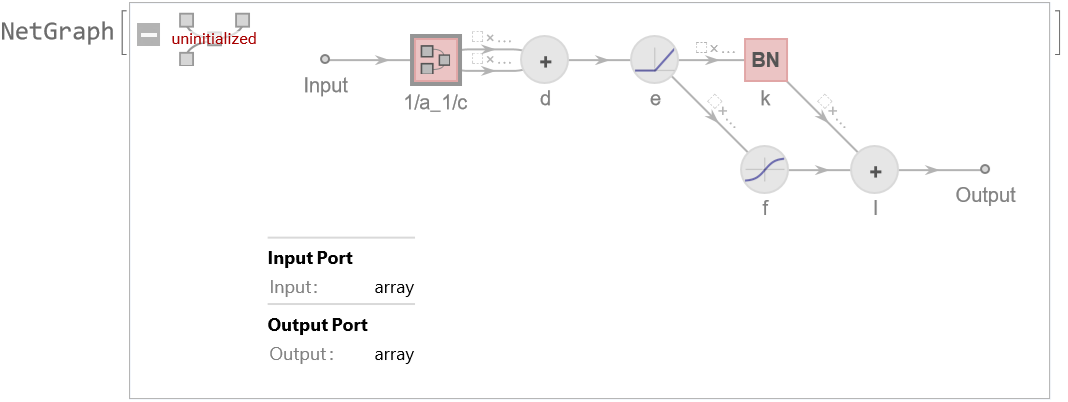Give a new name to the contracted group of vertices:

 In:=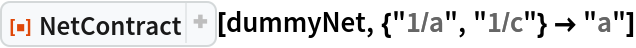Out=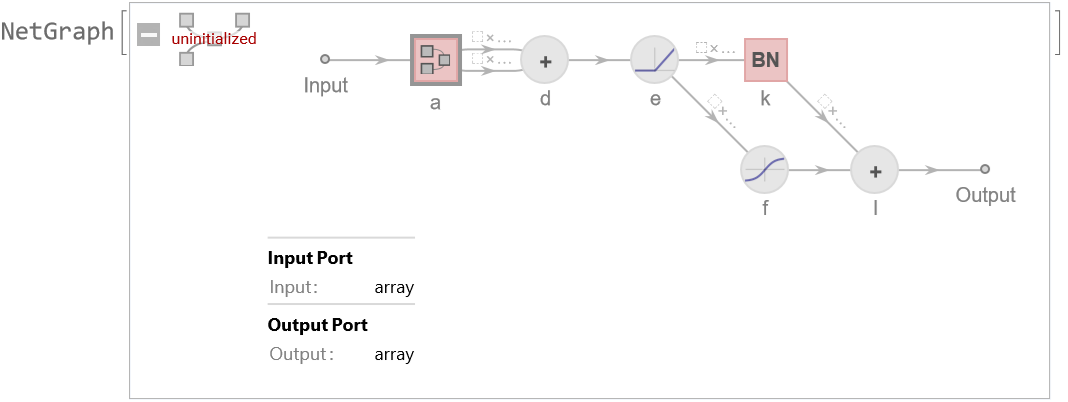Contract all the vertices after "1/a" and before "1/c":

 In:=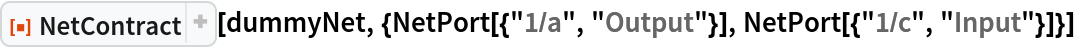Out=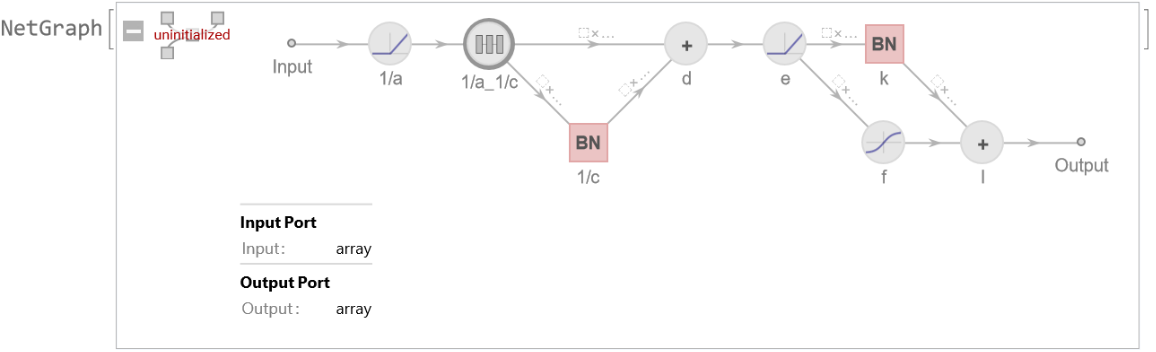In:=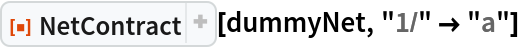Out=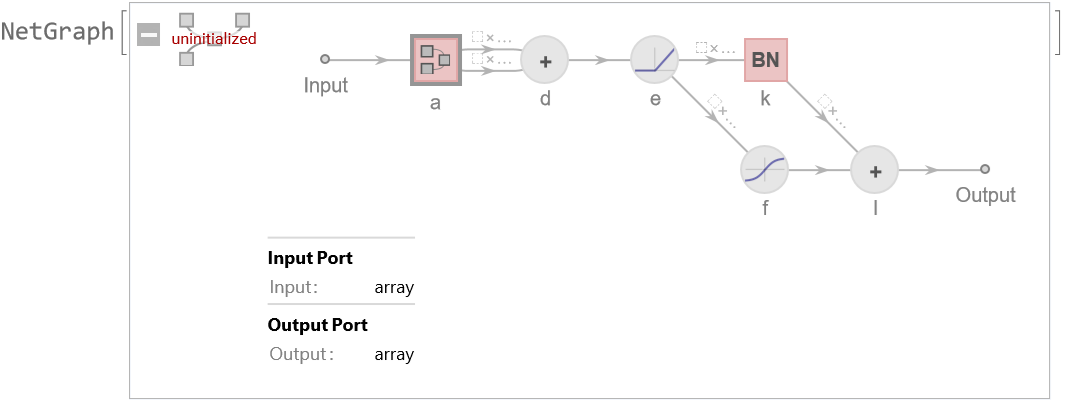Contract all the vertices which match a RegularExpression:

 In:=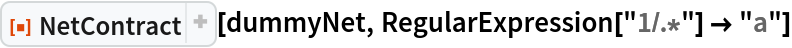Out=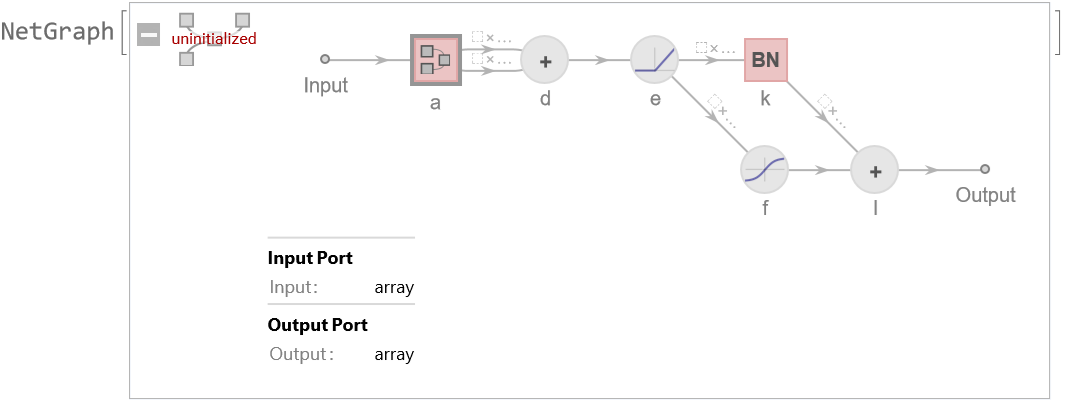Contract all the vertices which match a StringExpression:

 In:=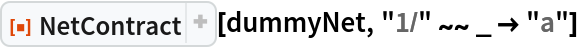Out=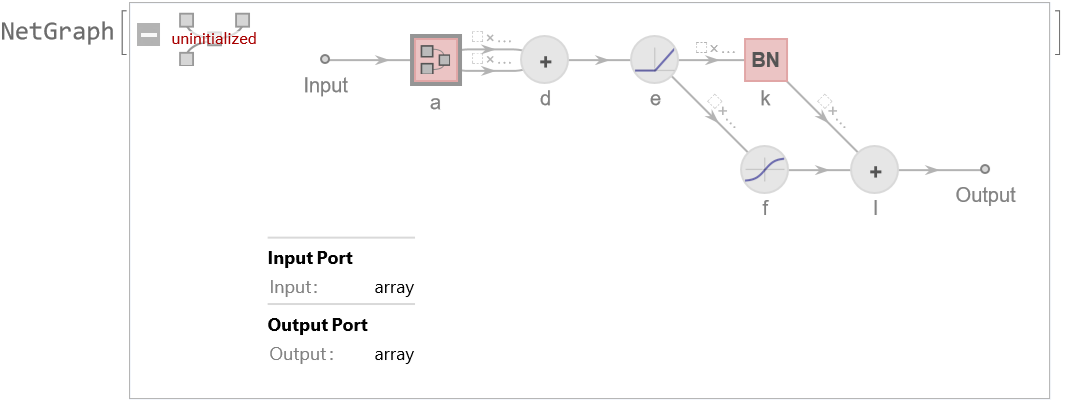Contract all the vertices which match a pure function:

 In:=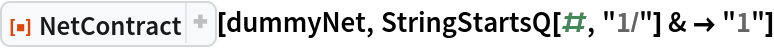Out=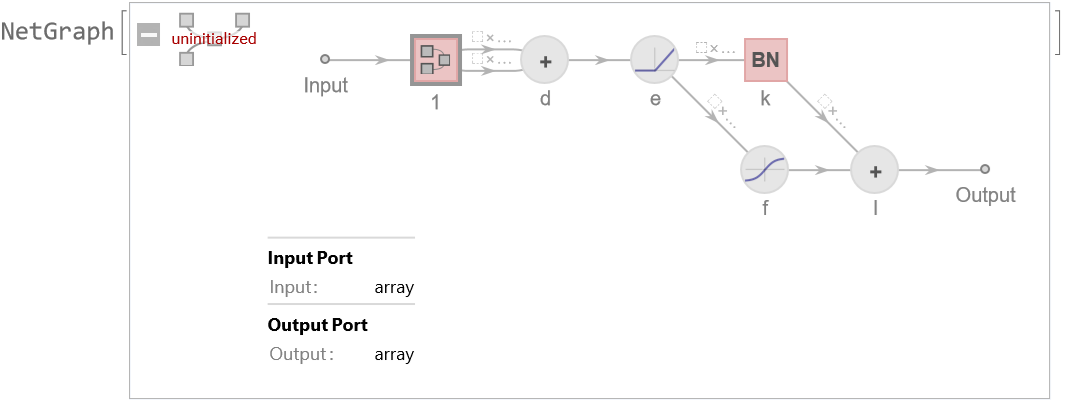Contract several sets of vertices:

 In:=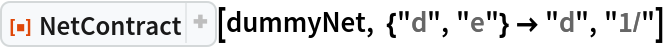Out=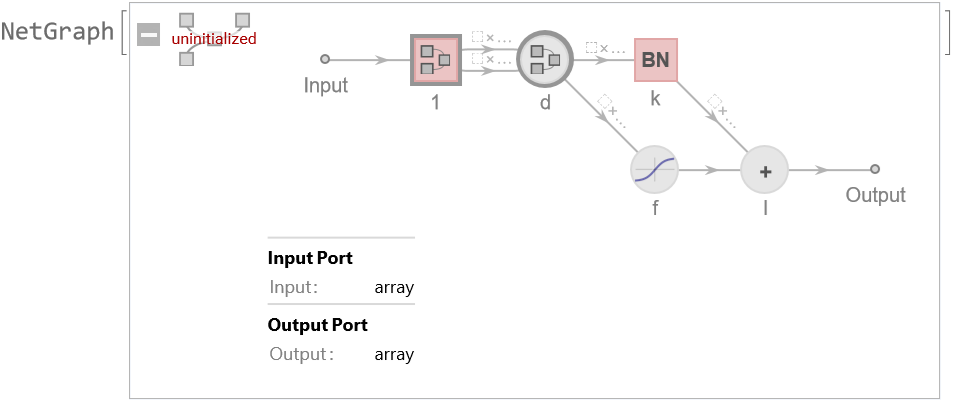### Scope (3)

Simplify the net by combining ThreadingLayer and LogisticSigmoid into a single layer:

 In:=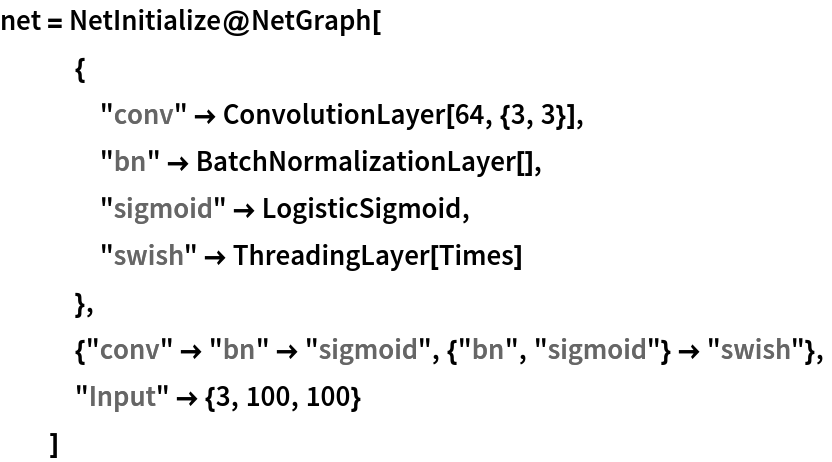Out=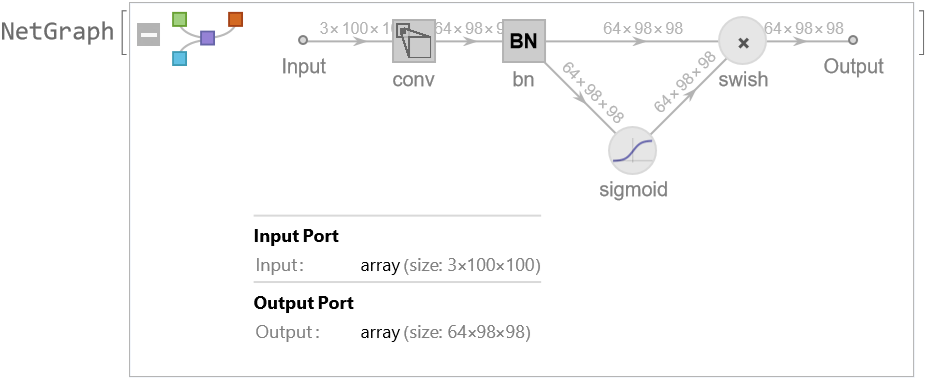Create a new net:

 In:=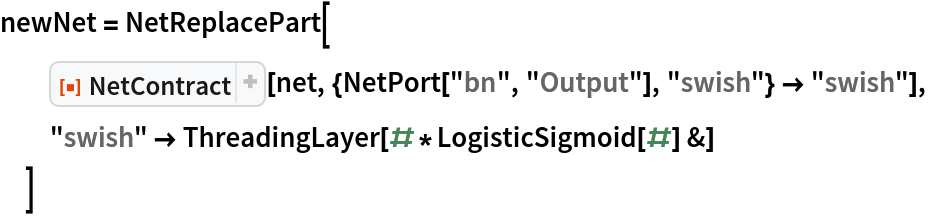Out=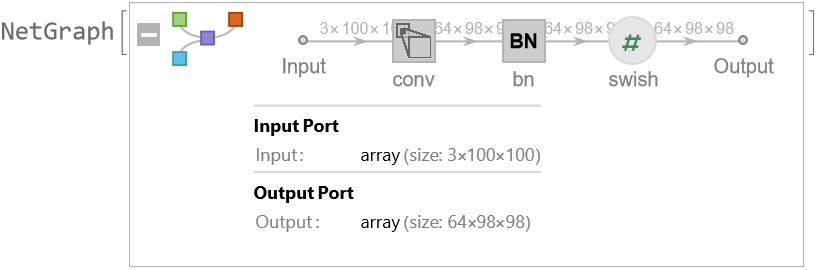Note that the new net is a bit faster:

 In:=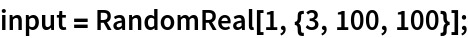In:=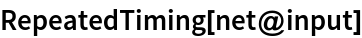Out=In:=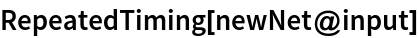Out=## Version History

• 1.0.0 – 01 November 2022